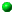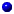### EXACT GEOMETRIC COMPUTATION:RealExpr (by Chee Yap, Tom Dube and Kouji Ouchi)
Real Expr Package -- original implementation of Core Library!EXACUS-webdemo
This great web-demo allows to you to input arbitrary bivariate polynomials, and it will analyse and render the corresponding real plane algebraic curves.Experimental Data and Code
for the paper
``Adaptive Isotopic Approximation of Nonsingular Curves: the parametric and nonlocal isotopy approach'',

Discrete & Comp.Geometry, 45(4)2011, pp.760-795 (also, 25th SoCG 2009).Classroom Examples of Nonrobust Geometric Computation
a resource page of source code, data and images based on our paper by this name. University of Tokyo. -->GeometryFactory Core Library is now distributed as one of GeometryFactory's exact number typesQuickMul (by Chee Yap and Chen Li)
Notes on a practical fast multiplication algorithm. The implementation is simple and direct. It will work for numbers up to 32 Megabytes. Rules of the game: (1) use FFT but no recursive call to multiplication allowed; (2) assume 32-bit machine arithmetic. Download of an implementation. Experimental results compared to GNU's gmp and CLN are described.Eigensolve: a polynomial root solver
A fast algorithm for complex root approximation from Steve Fortune at Bell Labs.Digital Library of Mathematical Functions (DLMF)
from NIST. ``The digital Abraham and Stegun''. Content.The Finite Element CircusMathematical Constants and Transcendental Functions:Xavier Gourdon and Pascal Sebah's page on Mathematical Constants, fast algorithms for their computation, programs, etc.Steven Finch's Essays on Mathematical Constants.Christian Gut's Tutorial on Hypergeometric Functions.Interval Methods and IEEE ArithmeticInterval Computation WebsiteIEEE Numerical Computation GuideValid LabPeopleRichard BrentDavid HoughSimon Langley has a page on the Uniformity Conjecture of Daniel RichardsonNorbert Mueller at University of Trier.Jean-Michel Muller at Ecole Normale Superiere, Lyon.Maurice Rojas at TAMUAbbas Edalat at Imperial CollegeJava Numerics: working group in Java Grande ForumSciMark 2.0: Java numerical benchmark. Others may be found at the javaNumerics page.Java Ultimate Math Package (JUMP): provides smooth transition from native datatypes and JDK number types to JUMP classes. JUMP Homepage.Meshing and TriangulationCart3D Package: A high-fidelity package for automated analysis of inviscid flows in complex geometric models from NASA Ames. Cartesian meshing is used.Triangulation and Meshing Packages: GNU Triangulated Surface (GTS) Library from sourceforge. The GTS Library has many basic models.Mesh Viewer: a lightweight application for viewing triangular meshes using the OpenGL API to render models from various file formats. Developed by Helmut Cantzler.Major Software LibrariesLEDA Project: A Library of Efficient Data Structures and Algorithms, from the Max-Planck Institute of Computer Science. Local Copy of the Web ManualAlgorithmic Solutions: an offshoot of the LEDA project.CGAL Library A Computational Geometry Algorithms Library.Geometry Factory an offshoot of the CGAL project.Big Number Packages and Software for Exact Computation:GNU MP Home: GNU's celebrated library for arbitrary precision arithmetic!PRECISE: Shankar Krishna's arbitrary precision library for reliable numeric and geometric computation.LiDIA Project: A C++ Library for Computational Number Theory.MPFUN Library: A multiprecision software package from David Bailey.CLN Package: A multiprecision software package from Bruno Haible.NTL from Victor Shoup. NTL is a portable C++ library providing data structures and algorithms for manipulating signed, arbitrary length integers, and for vectors, matrices, and polynomials over the integers and over finite fields.XR (Exact Real Arithmetic) from Keith Briggs, built on NTL.Floating Point Expansions: code and papers from Marc Daumas in the Arenaire Project (France).Exact Real Arithmetic from Abbas Edalat at Imperial College : domain theory viewpoint, applied to differential equations, etc.Online Bibliography List from SUNY Stony Brook (Comp.Sci. Bibliographies, Geom.bib, etc)Patent on efficient and reliable evaluation of mathematical functionsSoftware Development Tools:SUN Workshop Documentation: Compilers and software tools from SUN.VALGRIND: memory debugger from KDE development